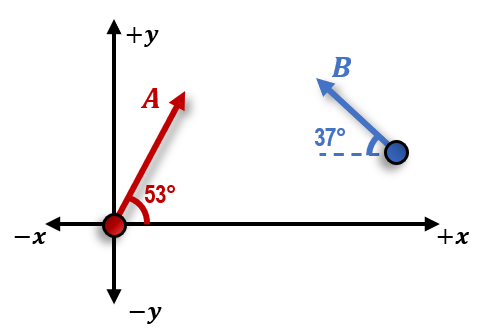Clutch Prep is now a part of Pearson
Ch 11: Momentum & ImpulseWorksheetSee all chapters

# Intro to Conservation of Momentum

See all sections
Sections
Intro to Momentum
Intro to Impulse
Impulse with Variable Forces
Intro to Conservation of Momentum
Push-Away Problems
Types of Collisions
Completely Inelastic Collisions
Adding Mass to a Moving System
Collisions & Motion (Momentum & Energy)
Ballistic Pendulum
Collisions with Springs
Elastic Collisions
How to Identify the Type of Collision
Intro to Center of Mass

Concept #1: Total Momentum of a System of Objects

Practice: Object A moves at 10 m/s at 53° and Object B moves at 5 m/s at –37° as shown below. Calculate the magnitude of the system’s total momentum if both objects have a mass of 2kg.Concept #2: Conservation Of Momentum

Practice: On a frictionless air hockey table, puck A of mass 0.250 kg moves to the right and collides with puck B of mass 0.38kg, which is initially at rest. After the collision, puck A is moving the left at 0.12 m/s and puck B moves to the right at 0.65 m/s. What was the initial velocity of puck A before the collision?